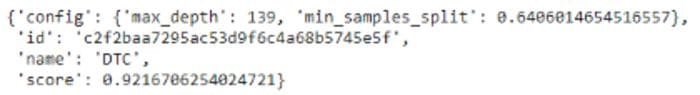Topics: AI | Data Science | Data Visualization | Deep Learning | Machine Learning | NLP | Python | R | Statistics

KDnuggets Home » News » 2021 » Mar » Tutorials, Overviews » Automating Machine Learning Model Optimization ( 21:n12 )

# Automating Machine Learning Model Optimization

This articles presents an overview of using Bayesian Tuning and Bandits for machine learning.

By Himanshu Sharma, Bioinformatics Data AnalystPhoto by Hunter Harritt on Unsplash

Creating a machine learning model is a difficult task because we need to make a model which works best for our data and we can optimize for better performance and accuracy. Generally making a machine learning model is easy but find out the best parameters and optimizing is a time taking process.

There are certain libraries/packages which allow you to automate this process and make machine learning models effortlessly. We can use these packages to select the best model for our data and also the best parameters for the model.

In this article, we will be discussing Bayesian tuning and bandits (BTB) which is used to select and hyperparameter tune the best model to solve a given machine learning problem. We will explore different functionalities that are provided for BTB for machine learning.

Let’s get started…

### Installing Required Libraries

In this article, we will be using Google Colab. Let’s install the required library i.e, BTB by using the command given below

``!pip install baytune``

### Importing Required Libraries

In this step, we will import all the required libraries that are sklearn, btb, etc.

``````from sklearn.datasets import load_breast_cancer
from sklearn.linear_model import SGDClassifier
from sklearn.metrics import f1_score, make_scorer
from sklearn.model_selection import cross_val_score
from sklearn.tree import DecisionTreeClassifier, ExtraTreeClassifier
from btb.tuning import Tunable
from btb.tuning import hyperparams as hp
from btb import BTBSession``````

Next, we will import the famous breast cancer dataset from sklearn and we will also define a dictionary that will contain the name of a different model that we want.

``````dataset = load_breast_cancer()
models = {
'DTC': DecisionTreeClassifier,
'SGDC': SGDClassifier,
'ETC': ExtraTreeClassifier,
}``````

### Calculating Score of Different Models

After defining the model names next we will create a function that will be used to calculate the scores of different models. We will use this function to compare the scores of different models.

``````def scoring_function(model_name, hyperparameter_values):
model_class = models[model_name]
model_instance = model_class(**hyperparameter_values)
scores = cross_val_score( estimator=model_instance,
X=dataset.data,y=dataset.target,
scoring=make_scorer(f1_score, average='macro'))
return scores.mean()``````

### Defining Tunable Hyperparameters

In this step we will define the hyperparameters which we want to tune, we will provide different hyperparameters for different models with range.

``````tunables = {
'DTC': Tunable({
'max_depth': hp.IntHyperParam(min=3, max=200),
'min_samples_split': hp.FloatHyperParam(min=0.01, max=1)
}),
'ETC': Tunable({
'max_depth': hp.IntHyperParam(min=3, max=200),
'min_samples_split': hp.FloatHyperParam(min=0.01, max=1)
}),
'SGDC': Tunable({
'max_iter': hp.IntHyperParam(min=1, max=5000, default=1000),
'tol': hp.FloatHyperParam(min=1e-3, max=1, default=1e-3),
}),
}``````

### Creating a BTB Session

Now, this is the Final Step we will create a BTB session by passing the tunable hyperparameters and the scoring function we define above to find out which model works best for our data.

``````session = BTBSession(
tunables=tunables,
scorer=scoring_function
)``````

Now after defining the session we will run this session by passing the number of iterations that we want.

``best_proposal = session.run(20)``

Now we will print out the result which is a dictionary containing the name of the best model along with the best hyperparameters values for that model.

``best_proposal``Best Model (Source: By Author)

This is how you can use BTB for selecting the best performing machine learning model with the best hyperparameter values. Go ahead try this with different datasets and let me know your comments in the response section.

### Before You Go

Thanks for reading! If you want to get in touch with me, feel free to reach me on hmix13@gmail.com or my LinkedIn Profile. You can view my Github profile for different data science projects and packages tutorials. Also, feel free to explore my profile and read different articles I have written related to Data Science.

Bio: Himanshu Sharma is a Bioinformatics Data Analyst at MEDGENOME. Himanshu is a Data Science Enthusiast with hands-on experience in analysing datasets, creating machine learning and deep learning models. He has worked on creating different data science projects and Poc's for different organisations. He has vast experience in creating CNN models for Image recognition and object detection along with RNN for time series prediction. Himanshu is an active blogger and have published around 100+ articles in the field of Data Science.

Original. Reposted with permission.

Related:

Top Stories Past 30 Days
Most Popular
Most SharedGet KDnuggets, a leading newsletter on AI, Data Science, and Machine Learning